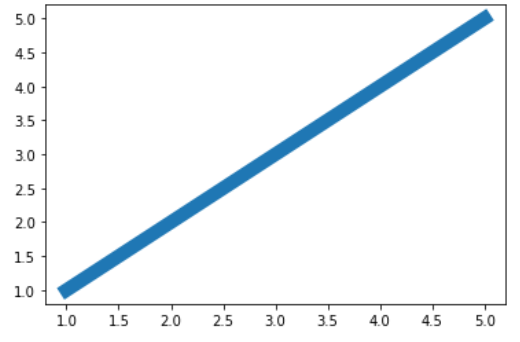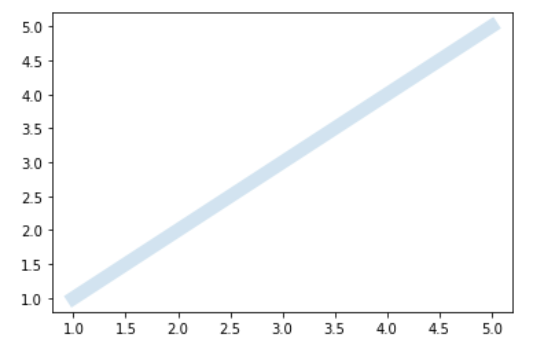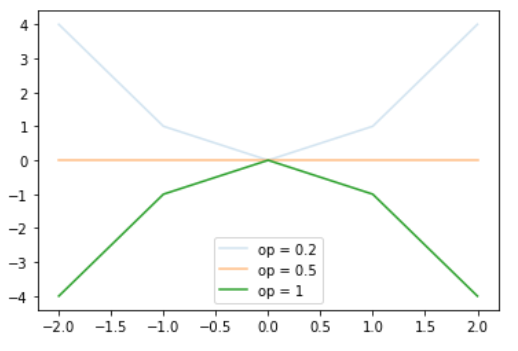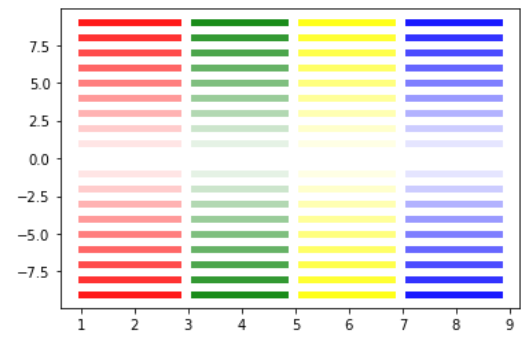# Change the line opacity in Matplotlib

• Last Updated : 17 Dec, 2020

In this article, we will learn how to Create the line opacity in Matplotlib. Let’s discuss some concepts :

• A line chart or line graph may be a sort of chart which displays information as a series of knowledge points called ‘markers’ connected by line segments. Line graphs are usually wont to find relationships between two data sets on the different axis; as example X, Y.
• Matplotlib allows you to regulate the transparency of a graph plot using the alpha attribute.
• By default, alpha=1.
• If you would like to form the graph plot more transparent, then you’ll make alpha but 1, such as 0.5 or 0.25.
• If you would like to form the graph plot less transparent, then you’ll make alpha greater than 1. This solidifies the graph plot, making it less transparent and more thick and dense, so to talk .

Approach:

Attention geek! Strengthen your foundations with the Python Programming Foundation Course and learn the basics.

To begin with, your interview preparations Enhance your Data Structures concepts with the Python DS Course. And to begin with your Machine Learning Journey, join the Machine Learning - Basic Level Course

1. Import Library (Matplotlib)
2. Import / create data.
3. Plot a graph (line) with opacity.

Example 1: (Simple line graph with its opacity)

## Python3

 `# importing libraries ` `import` `matplotlib.pyplot as plt ` ` `  `# create data ` `x ``=` `[``1``, ``2``, ``3``, ``4``, ``5``] ` `y ``=` `x ` ` `  `# plot the graph ` `plt.plot(x, y, linewidth``=``10``, alpha``=``0.2``) ` `plt.show() `

Output :Normal view (without alpha or alpha =1)Edited view (with alpha = 0.2)

Example 2: (Lines with different opacities)

## Python3

 `# importing libraries ` `import` `matplotlib.pyplot as plt ` `import` `numpy as np ` ` `  `# create data ` `x ``=` `np.array([``-``2``, ``-``1``, ``0``, ``1``, ``2``]) ` `y1 ``=` `x``*``0` `y2 ``=` `x``*``x ` `y3 ``=` `-``x``*``x ` ` `  `# plot the graph ` `plt.plot(x, y2, alpha``=``0.2``) ` `plt.plot(x, y1, alpha``=``0.5``) ` `plt.plot(x, y3, alpha``=``1``) ` `plt.legend([``"op = 0.2"``, ``"op = 0.5"``, ``"op = 1"``]) ` `plt.show() `

Output :Example 3: (Multiple line plots with multiple opacity)

## Python3

 `# importing libraries ` `import` `matplotlib.pyplot as plt ` `import` `numpy as np ` ` `  `# create data ` `x ``=` `[``1``, ``2``, ``3``, ``4``, ``5``] ` ` `  `# plot ` `for` `i ``in` `range``(``10``): ` `    ``plt.plot([``1``, ``2.8``], [i]``*``2``, linewidth``=``5``, color``=``'red'``, alpha``=``0.1``*``i) ` `    ``plt.plot([``3.1``, ``4.8``], [i]``*``2``, linewidth``=``5``, color``=``'green'``, alpha``=``0.1``*``i) ` `    ``plt.plot([``5.1``, ``6.8``], [i]``*``2``, linewidth``=``5``, color``=``'yellow'``, alpha``=``0.1``*``i) ` `    ``plt.plot([``7.1``, ``8.8``], [i]``*``2``, linewidth``=``5``, color``=``'blue'``, alpha``=``0.1``*``i) ` `     `  `for` `i ``in` `range``(``10``): ` `    ``plt.plot([``1``, ``2.8``], [``-``i]``*``2``, linewidth``=``5``, color``=``'red'``, alpha``=``0.1``*``i) ` `    ``plt.plot([``3.1``, ``4.8``], [``-``i]``*``2``, linewidth``=``5``, color``=``'green'``, alpha``=``0.1``*``i) ` `    ``plt.plot([``5.1``, ``6.8``], [``-``i]``*``2``, linewidth``=``5``, color``=``'yellow'``, alpha``=``0.1``*``i) ` `    ``plt.plot([``7.1``, ``8.8``], [``-``i]``*``2``, linewidth``=``5``, color``=``'blue'``, alpha``=``0.1``*``i) ` `     `  `plt.show() `

Output :My Personal Notes arrow_drop_up
Recommended Articles
Page :